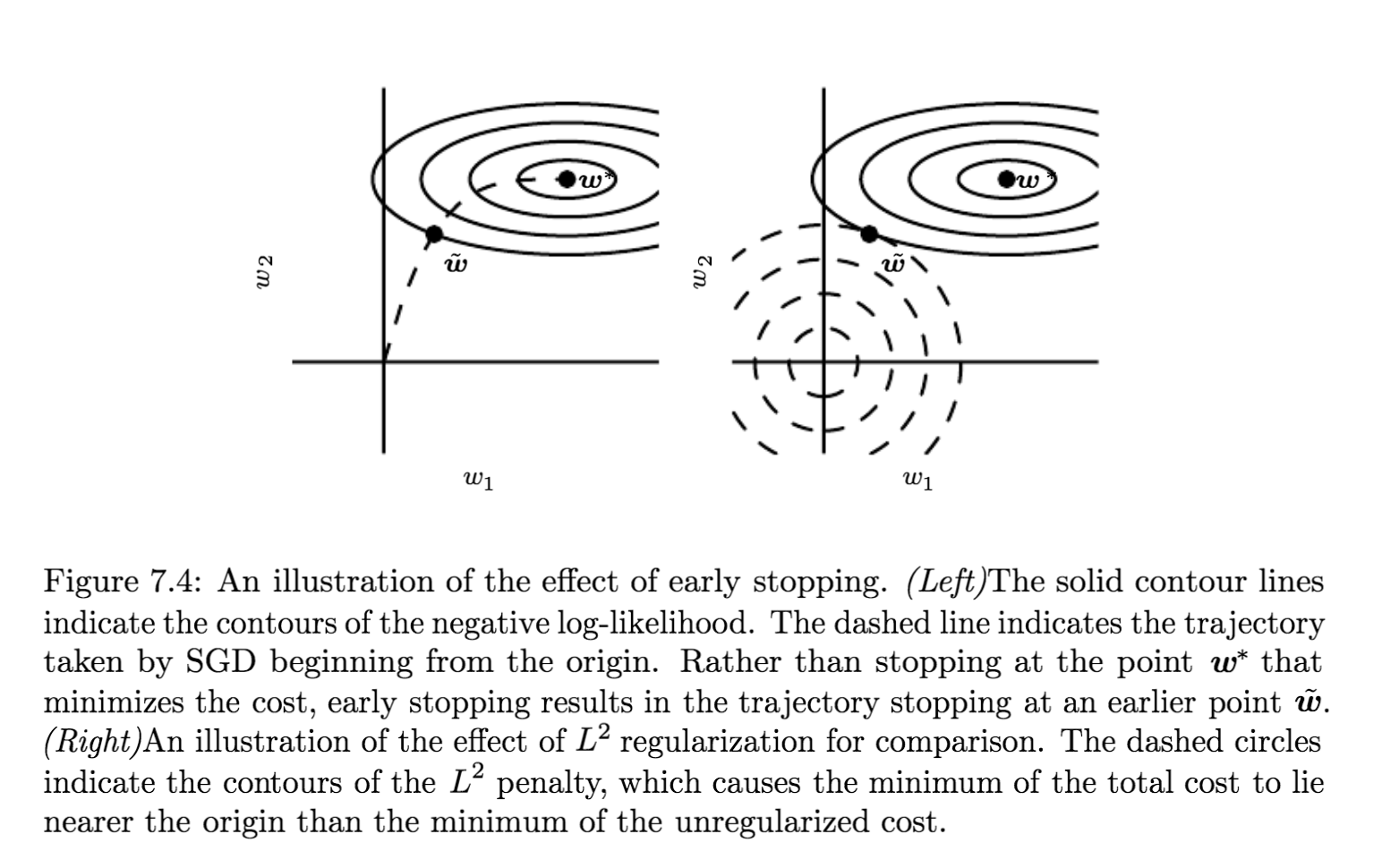# NIUHE

## Parameter Norm Penalties

### $L^2$ Parameter Regularzation

$L^2$ 正则化又叫 weight decay，岭回归（ridge regression）和 Tikhonov regularization。它在目标函数后加上惩罚项 $\Omega(\theta) = \frac{1}{2}||w||^2_2$，这使得权重趋近于向原点方向更新。

• $Q_a(a) = f(a)$
• $Q_a'(a) = f'(a)$
• $Q_a''(a) = f''(a)$

$\hat{J}$ 取得最小值时，它的梯度 $\bigtriangledown_w\hat{J}(w) = H(w - w^*)$ 等于 $0​$

$(H+\alpha I)\hat{w} = Hw^*$

$\hat{w} = (H+\alpha I)^{-1}Hw^*$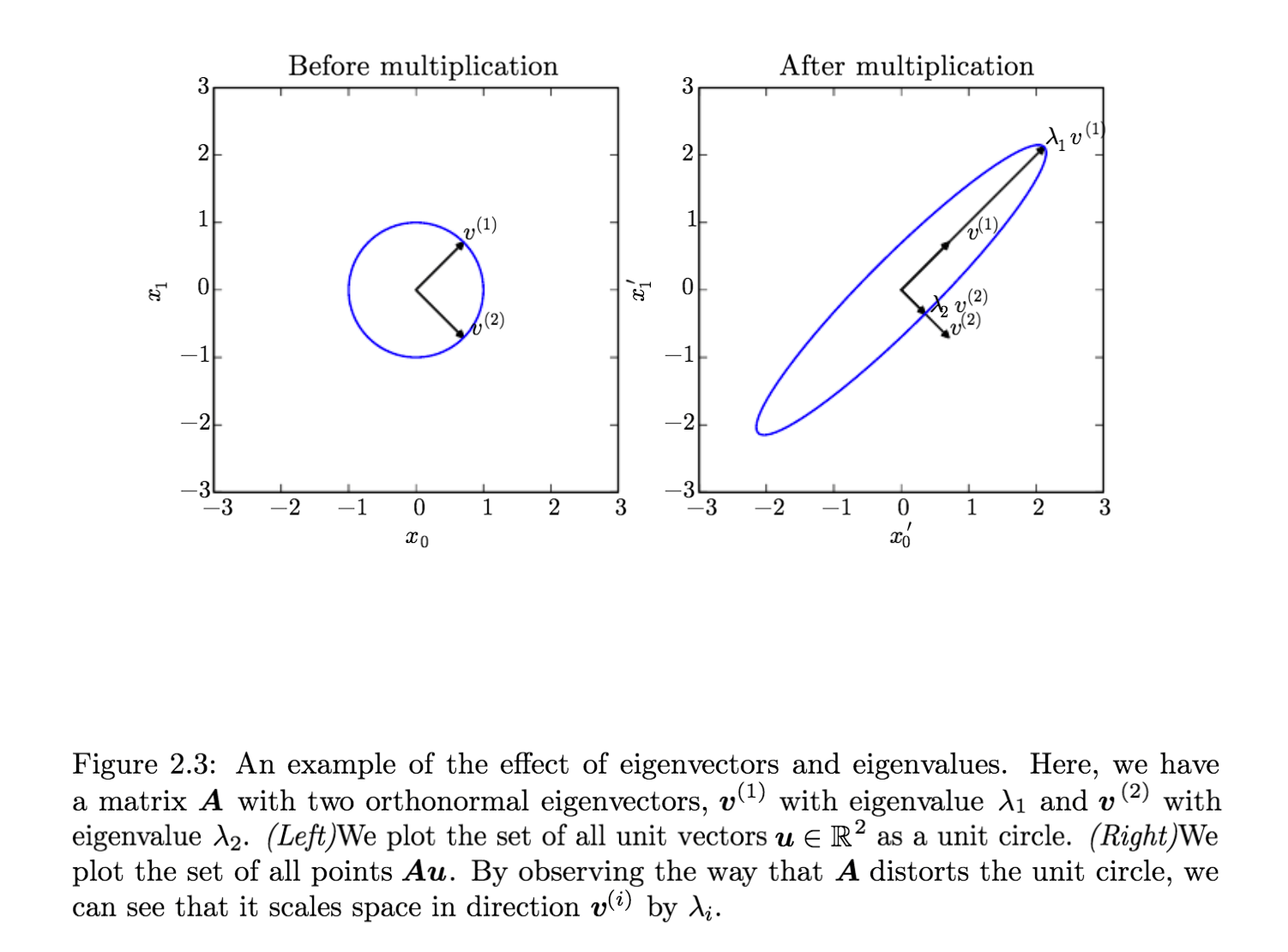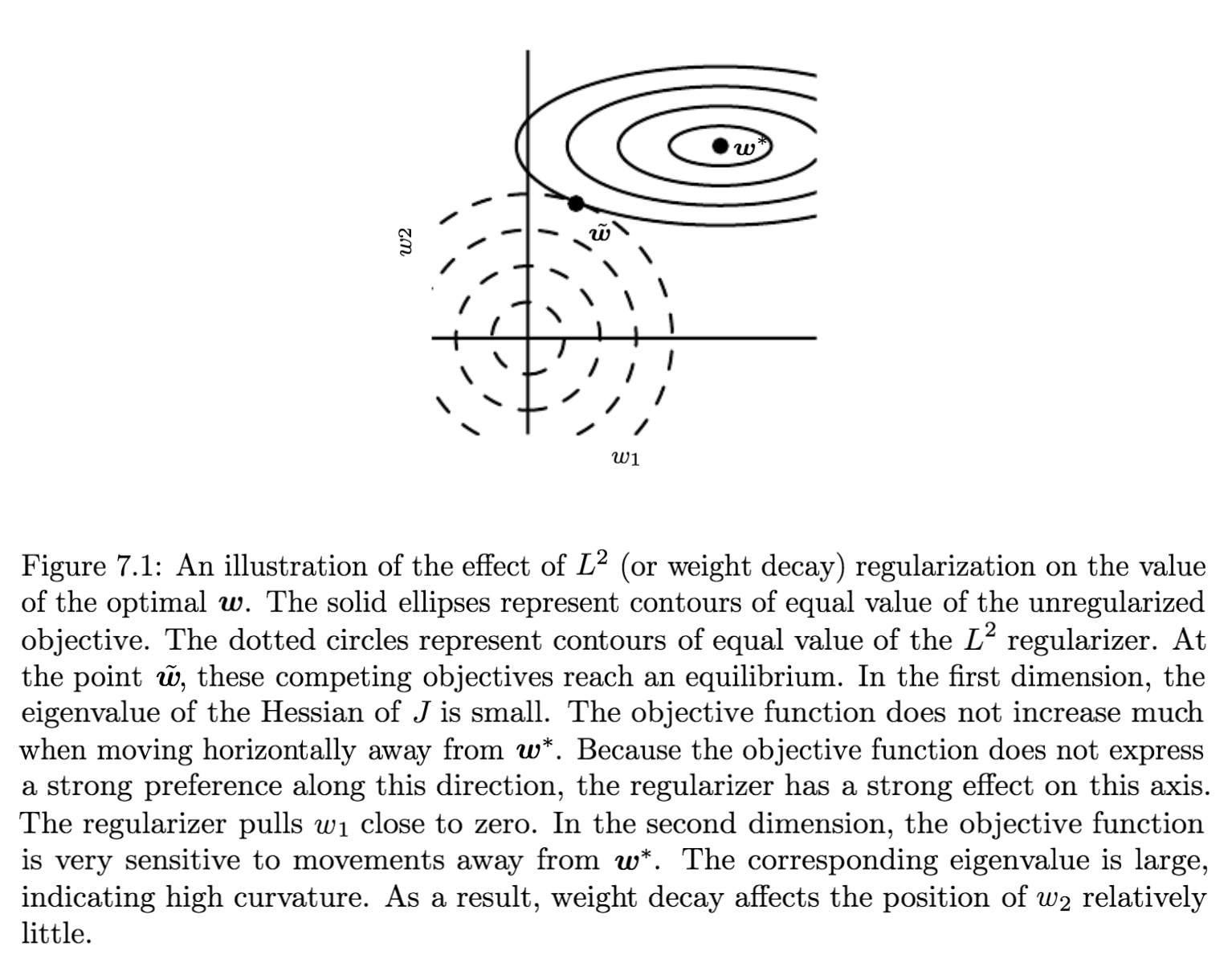### $L^1$ Regularization

1. $w_i^*≤\frac{\alpha}{H_{i,i}}$ 时，$w_i$ 的最优解为 $0$。This occurs because the contribution of $J(w;X,y)$ to the regularized objective $\hat{J}(w;X,y)$ is overwhelmed—in direction $i$ —by the $L^1$ regularization which pushes the value of $w_i$ to zero.
2. $w_i^*≥\frac{\alpha}{H_{i,i}}$ 时，这是最优的 $w_i$ 不会为 $0$，而是会向 $0$ 的方向移动 $\frac{\alpha}{H_{i,i}}$ 的距离。

$L^2$ 正则化相比，$L^1$ 正则化趋向于使权重变的稀疏（sparse），也就是说一部分参数的最优值为 $0$。如果我们用同样的假设分析 $L^2$ 正则化，则会得到 $\hat{w}_i = \frac{H_{i,i}}{H_{i,i}+\alpha}w^*_i$，只要 $w_i^*$ 非零，$\hat{w}_i$ 一定也非零。所以 $L^2$ 正则化不会使参数变稀疏，而 $L^1$ 正则化在 $\alpha$ 很大的情况下会。

$L^1$ 正则化的这个性质可以用来做特征选择（feature selection），如果某个特征对模型收敛帮助不大，则它对应的权重会收缩到 $0$，我们就可以去掉那些用处不大的特征。

Maximum A Posteriori (MAP) Estimation $\theta_{MAP} = \arg\max_\theta p(\theta|x) = \arg\max_\theta \log p(x|\theta)+\log p(\theta)$ Maximum Likelihood Estimator (MLE) $\theta_{ML} = \arg\max_\theta p(X;\theta)$

## Noise Robustness

Injecting Noise at the Output Targets

1. 任务相关的参数，只从任务相关的样本中优化参数，在下图中的上层
2. 一般的参数，不与任务相关，可以从所有样本中更新参数，在下图中的底层（$h^{(shared)}$）。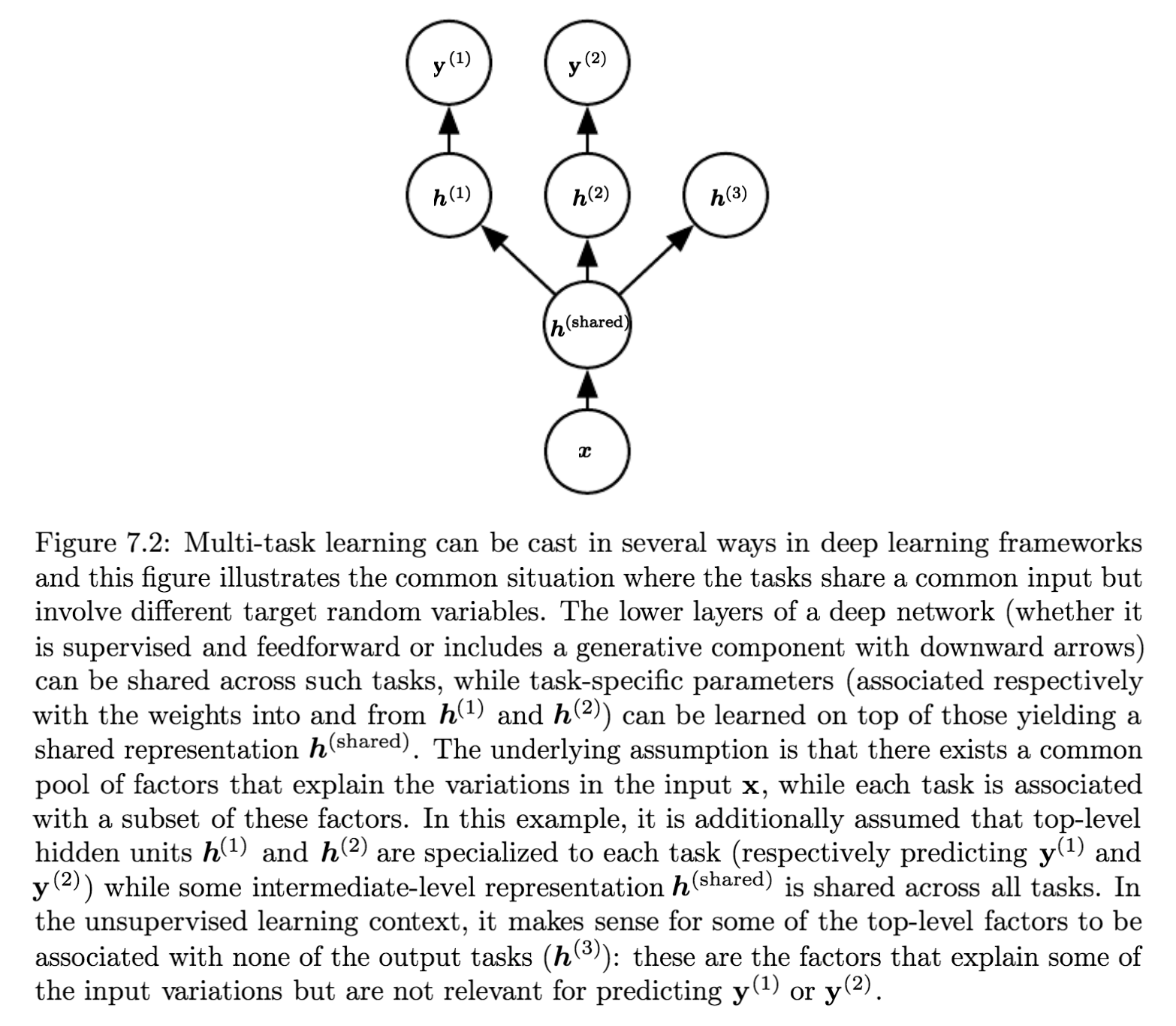## Early Stopping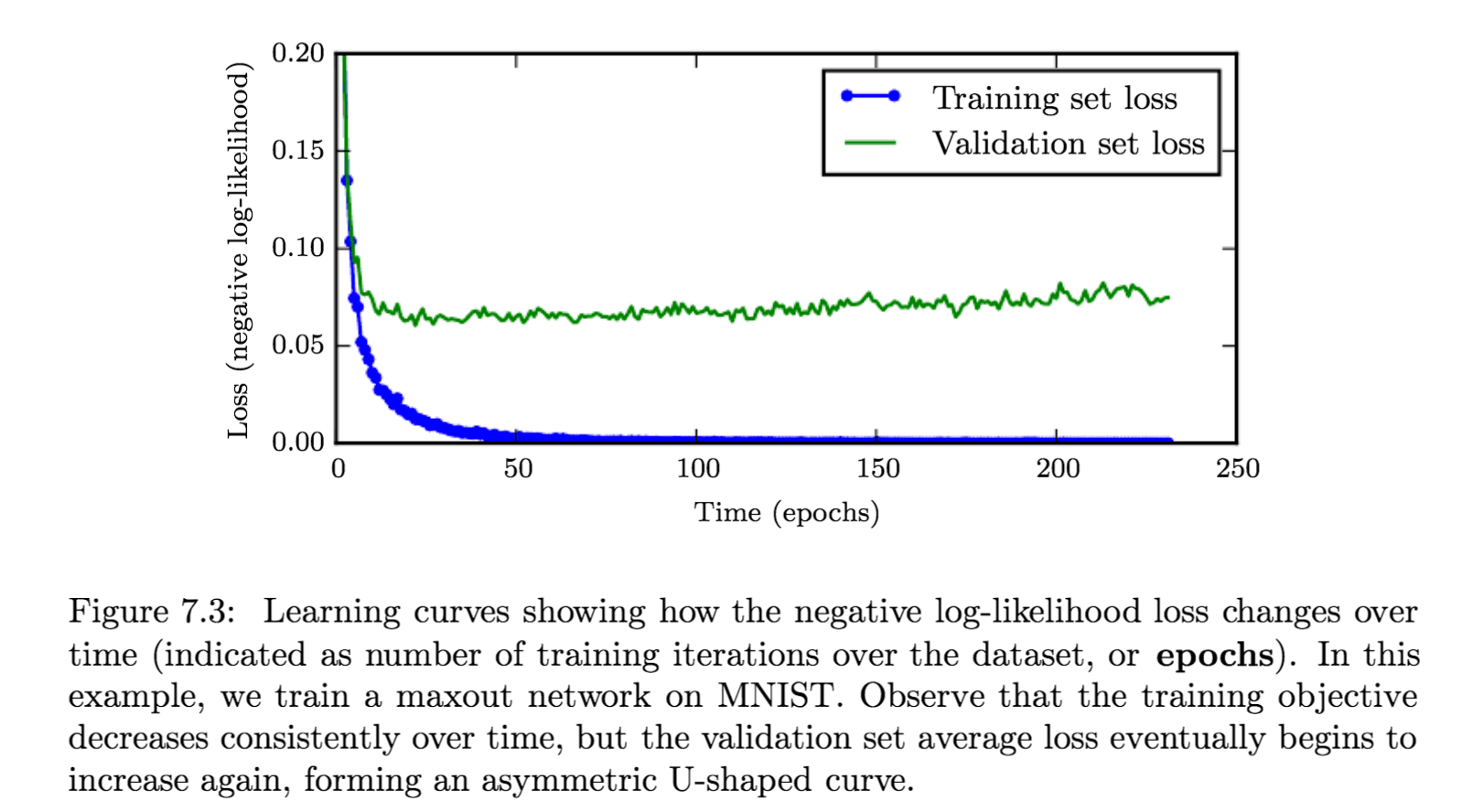Early stopping 策略是一种很常用正则化方法，因为它既有效又简单。它也可以看做是超参数选择的方法，因为训练的轮数也是个超参数，而应用 early stopping 策略就可以自动选择这个值，而不需要大量猜测。

How early stopping acts as a regularizer

Early stopping 会限制权重更新次数，即限制了权重（参数）远离初始权重的距离，如果最佳采取 $\tau$ 轮迭代，学习速率为 $\epsilon$ ，那么它俩的乘积 $\epsilon\tau$ 就类似于 $L^2$ 正则化中的系数！